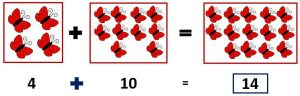An addition is adding the numbers of two or more numbers of objects by adding we get the sum of the numbers.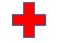means to put together or add.

Example:

When we add 1 to any Number, the answer is the next number in counting.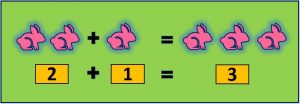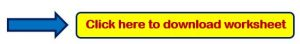Example 1: Add the following number?

### 3 + 2

####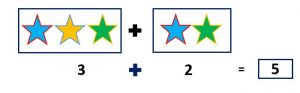Example 2: Add the following number?

### 6 + 3

####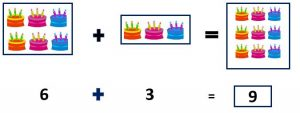Example 3: Add the following number?

### 4 +10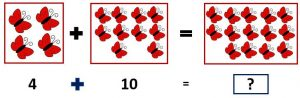#### Therefore,